# 1. Mole Balances*

Whenever the symbols below appear, audio is available
for use, starting with the ones below.

## Topics

1. Chemical Identity
2. Reaction Rate
3. General Mole Balance Equation
4. Mole Balance on Different Reactor Types
5. Self Test Exercises

 Chemical Identity top

A chemical species is said to have reacted when it has lost its chemical identity. The identity of a chemical species is determined by the kind, number, and configuration of that species' atoms.

Three ways a chemical species can lose its chemical identity:

1. decomposition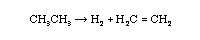2. combination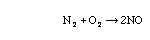3. isomerization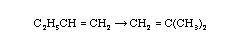Reaction Rate (p.2) top

The reaction rate is the rate at which a species looses its chemical identity per unit volume. The rate of a reaction can be expressed as the rate of disappearance of a reactant or as the rate of appearance of a product. Consider species A:

A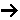B

rA = the rate of formation of species A per unit volume
-rA = the rate of a disappearance of species A per unit volume
rB = the rate of formation of species B per unit volume

Example: AB

If B is being created at a rate of 0.2 moles per decimeter cubed per second, ie, the rate of formation of B is,
rB = 0.2 mole/dm3/s

Then A is disappearing at the same rate:
-rA = 0.2 mole/dm3/s
the rate of formation of A is
rA = -0.2 mole/dm3/s

For a catalytic reaction, we refer to -rA', which is the rate of disappearance of species A on a per mass of catalyst basis.

NOTE: dCA/dt is not the rate of reaction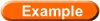Is sodium hydroxide reacting?

Consider species j:

• rj is the rate of formation of species j per unit volume [e.g. mol/dm3*s]
• rj is a function of concentration, temperature,pressure, and the type of catalyst (if any)
• rj is independent of the type of reaction system (batch, plug flow, etc.)
• rj is an algebraic equation, not a differential equation

We use an algebraic equation to relate the rate of reaction, -rA, to the concentration of reacting species (e.g., CA) and to the temperature (T) at which the reaction occurs [e.g. -rA = k(T)CA2].

Reaction Video: Sodium with ChlorineWould you like to see some other rates of reaction?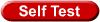The Convention for Rates of Reaction

 General Mole Balance Equation (p.6) top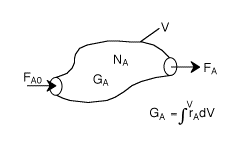FA0= Entering molar flow rate of A (mol/time)
FA= Exiting molar flow rate of A (mol/time)
GA= Rate of generation(formation) of A (mol/time)
V = Volume (vol e.g. m3)
rA= rate of generation(formation) of A (mole/time•vol)
NA= number of moles of A inside the system Volume V (mols)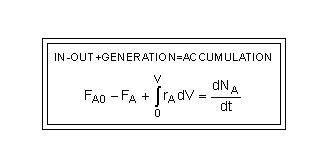How about testing what you've learned?

 Mole Balance on Different Reactor Types (p.25) top

The GMBE applied to the four major reactor types (and the general reaction, A->B):

Reactor Differential Algebraic Integral
Batch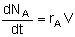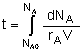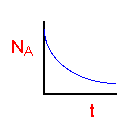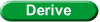CSTR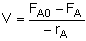PFR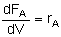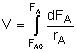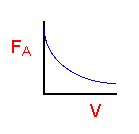PBR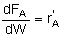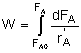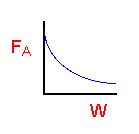Self Test Exercises topMultiple Choice QuestionsBatch Reactor TimeWhat's wrong with this solution?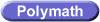Polymath ODE solver tutorialPolymath NLE solver tutorial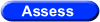Objective Assessment of Chapter 1

The following movie was made by the students of Professor Alan Lane's chemical reaction engineering class at the University of Alabama Tuscaloosa

* All chapter references are for the 4th Edition of the text Elements of Chemical Reaction Engineering .